Edumarz# Question3: Show how √𝟓 can be represented on the number line.

-Ushma Gaur, Subject Matter Expert at Edumarz

Solution:

Draw a number line and marking as point O and A at the place 0 and 1 on the drawn number line. Then draw BA of unit length perpendicular to OA. After that join O to B.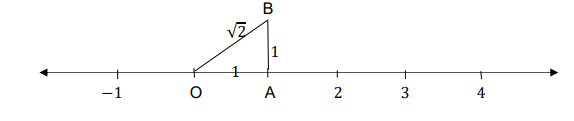In triangle OAB, by Pythagoras theorem, OB2=OA2+AB2

OB2=12+12=2

Using the compass with Centre O and radius OB, draw an arc intersecting the number line at the point P. Then P corresponds to 2 on the number line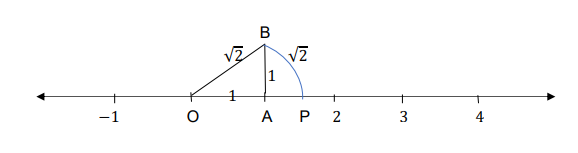Further, draw BD of unit length perpendicular to OB and join OD. Then using Pythagoras theorem, OD2=OB2+BD2

OD2=22+12=3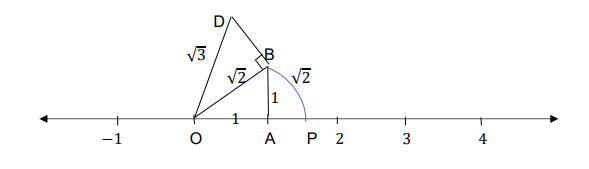Using the compass with centre O and radius OD, draw an arc intersecting the number line at the point Q. Then Q corresponds to 3 on the number line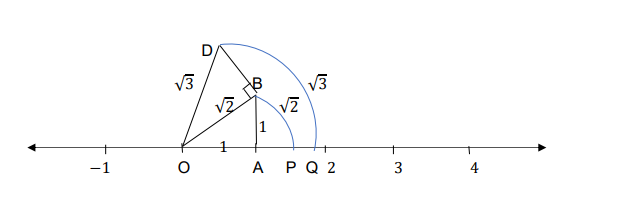Further, draw CD of unit length perpendicular to OD and join OC. Then using Pythagoras theorem

OC2=OD2+CD2

OC2=32+12=4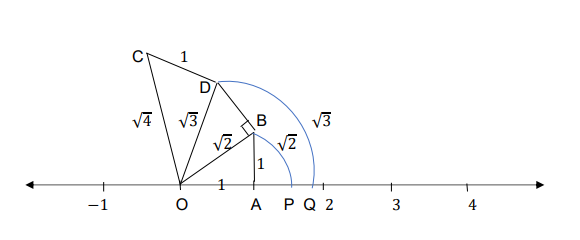Using the compass with centre O and radius OC, draw an arc intersecting the number line at the point R = 2 . Then R corresponds to4 on the number line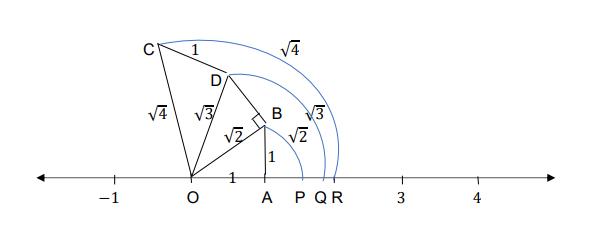Again, repeating the above process one more time to get 5

Draw ED of unit length perpendicular to OC and join OE. Then using Pythagoras theorem

OE2=OC2+CE2

OE2=42+12=5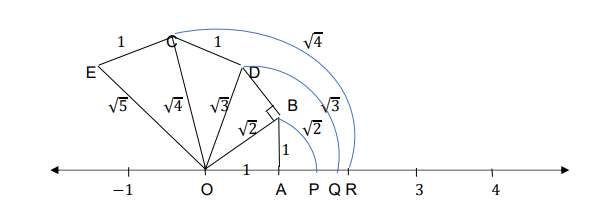Using the compass with centre O and radius OE, draw an arc intersecting the number line at the point S . Then S corresponds to 5 on the number line.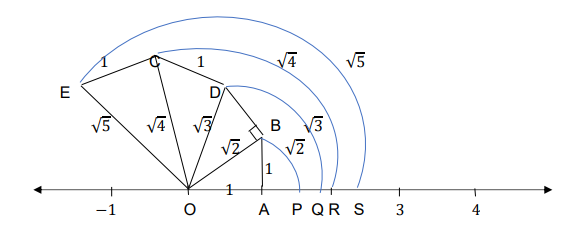## AMU, a top-notch opportunity## AIMS Test for Management## BANARAS HINDU UNIVERSITY